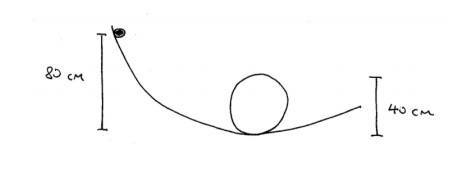# Loop-the-loop ball Physics question

fantisism

## Homework Statement

A 150 gram ball slides down a smooth track with no friction, which has a circular loop with radius 20 centimeters. The ball has an initial speed of 3 m/s. Its initial position is 80 centimeters above the lowest point of the circular loop. Calculate the normal force of the track on the ball when it is at the lowest point of the circular loop.n-mg=m(v2/r)

## The Attempt at a Solution

I have drawn a force diagram for when the ball is at the lowest point on the circular loop with n (normal force) pointing upwards and mg downwards. I THINK the only formula I need for this problem is what I already mentioned. I'm sure I substituted in most of the values right except for the v. I made v equal to 3 m/s but I'm sure it'll be faster when it's at the bottom of the circular loop. With v=3 m/s, m=0.15 kg, r=0.2 m, and g=9.8 m/s2, I got n to be equal to 8.22 N.

## Answers and Replies

Homework Helper
Gold Member
I'm sure it'll be faster when it's at the bottom of the circular loop
Yes.
Hint: Conservetion of energy...

fantisism
Yes.
Hint: Conservetion of energy...

Would I use v=sqrt(2gh) in this example?

Homework Helper
Gold Member
Would I use v=sqrt(2gh) in this example?
Right. Lost potential energy is converted into kinetic energy.

atom jana
The initial kinetic energy as well as the gravitational potential energy should be taken into account.

Homework Helper
Gold Member
Right.I didn't read the initial velocity part in the problem.

fantisism
The initial kinetic energy as well as the gravitational potential energy should be taken into account.
Right.I didn't read the initial velocity part in the problem.
So in order to find the velocity when the ball reaches the bottom of the loop, I would use 0.5*m*(vi)2+0.5*m*(vf)2=mgh and solve for vf?

Homework Helper
Gold Member
The expression would be 0.5*m*vf2-0.5*m*vi2=mgh.

fantisism
The expression would be 0.5*m*vf2-0.5*m*vi2=mgh.
So if I had to solve vf, it would be sqrt(2gh)+3 m/s?

Homework Helper
So if I had to solve vf, it would be sqrt(2gh)+3 m/s?
No.

Homework Helper
Gold Member
it would be sqrt(2gh)+3 m/s?
No. Rearrange the terms carefully.

fantisism
No.

No. Rearrange the terms carefully.
vf=sqrt(2gh+(vi)2)?

Homework Helper
Gold Member
vf=sqrt(2gh+(vi)2)?
Looks good!

fantisism
Looks good!
And then once I find that out, I would subtract vi from vf and use that value for v in n-mg=m(v2/r)?

Homework Helper
Gold Member
I would subtract vi from vf
Why?
n-mg=m(v2/r)?
v in this formula is nothing but your vf.

fantisism
Why?

v in this formula is nothing but your vf.

Oh alright then. I thought v in the n-mg=m(v2/r) was the change in velocity, although now that I think about it, it's unnecessary. Thank you so much!